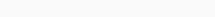# Math Puzzles Puzzle 43 Answer AndroidMath puzzle game level 43 solution with detailed hints and explanation, Scroll below to find out.

Math puzzles increase your logical thinking. The objective of the game is to find patterns hidden in the puzzle in order to arrive at the solution. Challenge yourself with different levels of math puzzles and stretch the limits of your intelligence.

### Math Puzzles Puzzle 43:

3 6 5 7 8 11 15 ?Solution/Hint: Every number is the sum of the previous 2 number minus 4

15+11-4=22

If there is any Doubt or you are unable to understand the solution, then please let me know in comments, I will surely help you.

### 2 thoughts on “Math Puzzles Game Level 43 Answer with Solution”

•February 28, 2021 at 11:37

Level 43
7,3=32
10,6=77
8,2=27
5,7=?
What is the answer in this question

•